GFG App
Open AppBrowser
Continue

# Set Axis Limits of ggplot2 Facet Plot in R – ggplot2

In this article, we will discuss how to set the axis limits of the ggplot2 facet plot in the R programming language.

## Method 1: Set axis limits of ggplot2 facet plot with Free Scales

Here the role of the ggplot2 package is to plot the facet plot and provide some functionalities to the user, further the user needs to set the argument of the scales function to “free” this will be freely set the axis limits of the facet ggplot2 plot.

Scale function:

This is a generic function whose default method centers and/or scales the columns of a numeric matrix.

Syntax: scale(x, center = TRUE, scale = TRUE)

Parameters:

• x: a numeric matrix
• center: either a logical value or numeric-alike vector of length equal to the number of columns of x
• scale: either a logical value or a numeric-alike vector of length equal to the number of columns of x.

Example:

In this example, we will be looking at the facet plot created with 100 random data points and then with the help of the free scaling method, we will be limiting the axis of the facet ggplot2 in the R programming language.

Initial plot with setting the axis limits: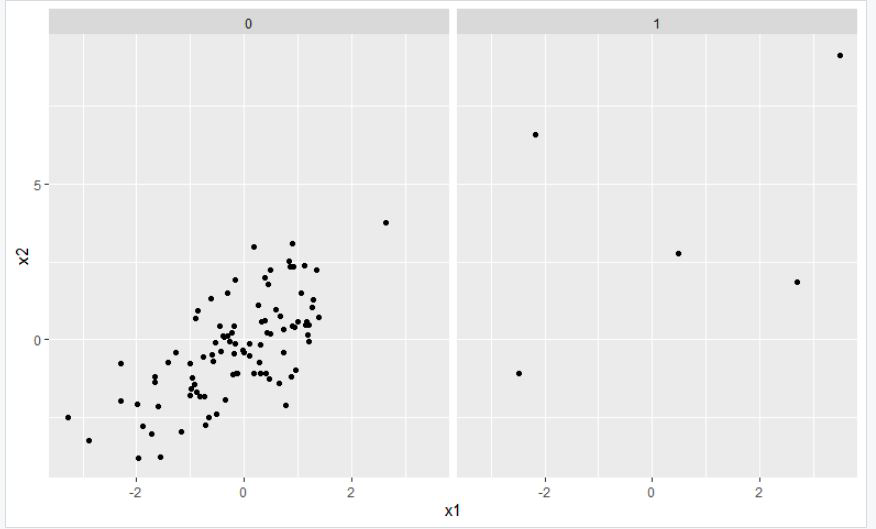Code:

## R

 `# load ggplot2 ` `library``(``"ggplot2"``) ` ` `  `# Data from the facet plot ` `x1 < -``rnorm``(100) ` `x2 < -``rnorm``(100)+x1 ` `grp < - ``rbinom``(100, 1, 0.1) ` ` `  `x1[grp == 1] < - x1[grp == 1] * 5 ` `x2[grp == 1] < - x2[grp == 1] * 5 ` ` `  `# Data from the facet plot ` `gfg < - ``data.frame``(x1, x2, grp) ` ` `  `# facet plot with facet_wrap ` `gfg_plot < - ``ggplot``(gfg, ``aes``(x1, x2)) + ` `geom_point``() + ``facet_wrap``(~ grp) ` ` `  `# Draw plot with free scales ` `gfg_plot + ``facet_wrap``(~ grp, scales=``"free"``) `

Output: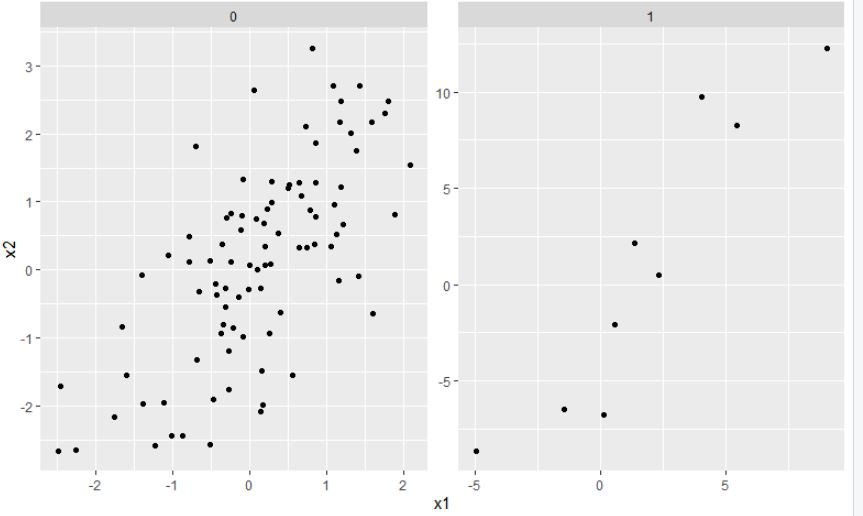## Method 2: Set axis limits of ggplot2 facet plot with Free X-axis

Here the role of the ggplot2 package is to plot the facet plot and provide some functionalities to the user, further the user needs to set the argument of the scales function to “free_x” this will be freely set the axis limits of x-axis of the facet ggplot2 plot and the remaining y-axis will be unchanged.

Example:

In this example, we will be looking at the facet plot created with 100 random data points and then with the help of the free X-axis scaling method, where the x-axis of the plot with be changing the limits and the remaining y-axis will be unchanged the facet ggplot2 in the R programming language.

Initial plot with setting the axis limits:Code:

## R

 `# load ggplot2 ` `library``(``"ggplot2"``) ` ` `  `# Data from the facet plot ` `x1 < -``rnorm``(100) ` `x2 < -``rnorm``(100)+x1 ` `grp < - ``rbinom``(100, 1, 0.1) ` `x1[grp == 1] < - x1[grp == 1] * 5 ` `x2[grp == 1] < - x2[grp == 1] * 5 ` ` `  `# Data from the facet plot ` `gfg < - ``data.frame``(x1, x2, grp) ` ` `  `# facet plot with facet_wrap ` `gfg_plot < - ``ggplot``(gfg, ``aes``(x1, x2)) + ` `geom_point``() + ``facet_wrap``(~ grp) ` ` `  `# Draw plot with free x-axis scales ` `gfg_plot + ``facet_wrap``(~ grp, scales=``"free_x"``) `

Output: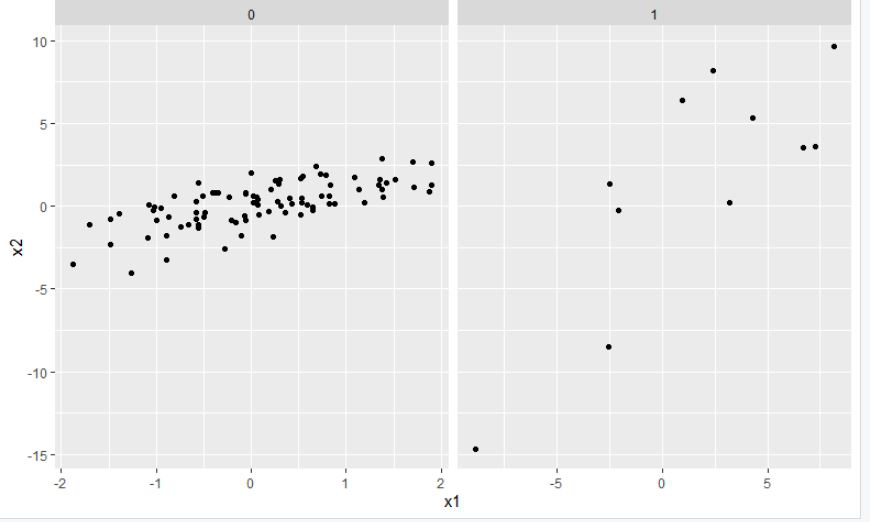## Method 3: Set axis limits of ggplot2 facet plot with Free Y-axis

Here the role of the ggplot2 package is to plot the facet plot and provide some functionalities to the user, further the user needs to set the argument of the scales function to “free_y” this will be freely set the axis limits of y-axis of the facet ggplot2 plot and the remaining x-axis will be unchanged.

Example:

In this example, we will be looking at the facet plot created with 100 random data points and then with the help of the free Y-axis scaling method, where the y-axis of the plot with be changing the limits and the remaining x-axis will be unchanged the facet ggplot2 in the R programming language.

Initial plot with setting the axis limits:Code:

## R

 `# load ggplot2 ` `library``(``"ggplot2"``) ` ` `  `# Data from the facet plot ` `x1 < -``rnorm``(100) ` `x2 < -``rnorm``(100)+x1 ` `grp < - ``rbinom``(100, 1, 0.1) ` ` `  `x1[grp == 1] < - x1[grp == 1] * 5 ` `x2[grp == 1] < - x2[grp == 1] * 5 ` ` `  `# Data from the facet plot ` `gfg < - ``data.frame``(x1, x2, grp) ` ` `  `# facet plot with facet_wrap ` `gfg_plot < - ``ggplot``(gfg, ``aes``(x1, x2)) + ` `geom_point``() + ``facet_wrap``(~ grp) ` ` `  `# Draw plot with free y-axis scales ` `gfg_plot + ``facet_wrap``(~ grp, scales=``"free_y"``) `

Output: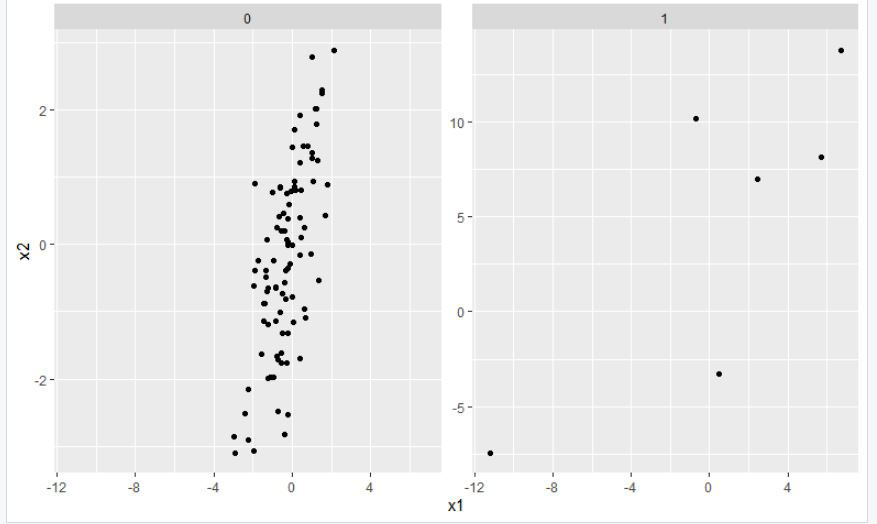## Method 4: Set axis limits of ggplot2 facet plot with Individual Axes

Here, the user needs to set the argument of the scales function to “free_x” this will be freely set the axis limits of y-axis of the facet ggplot2 plot and the remaining x-axis will be changed using the ylim function which is the manual setting up the plot axis .

Example:

In this example, we will be looking at the facet plot created with 100 random data points and then with the help of the free X-axis scaling method, where the x-axis of the plot with be changing the limits and the remaining y-axis will be changed  with the help of the ylim() function the facet ggplot2 in the R programming language.

Initial plot with setting the axis limits:-Code:

## R

 `# load ggplot2 ` `library``(``"ggplot2"``)   ` ` `  `# Data from the facet plot ` `x1<-``rnorm``(100)             ` `x2<-``rnorm``(100)+x1 ` `grp <- ``rbinom``(100, 1, 0.1) ` `x1[grp == 1] <- x1[grp == 1] * 5 ` `x2[grp == 1] <- x2[grp == 1] * 5 ` ` `  `# Data from the facet plot ` `gfg <- ``data.frame``(x1,x2, grp)       ` ` `  `# facet plot with facet_wrap ` `gfg_plot <- ``ggplot``(gfg, ``aes``(x1,x2)) +             ` `geom_point``() +  ``facet_wrap``(~ grp) ` `gfg_plot + ``facet_wrap``(~ grp, scales = ``"free_x"``)+``coord_cartesian``(ylim = ``c``(-20,20)) `

Output: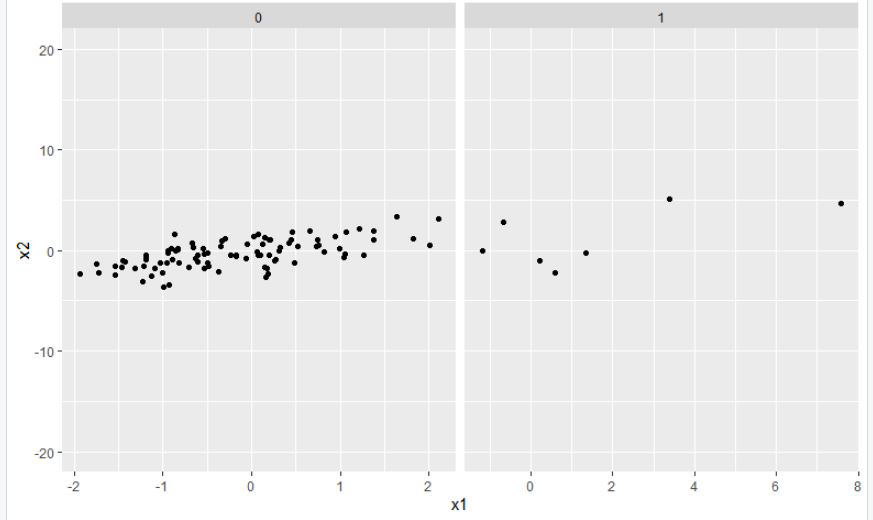My Personal Notes arrow_drop_up